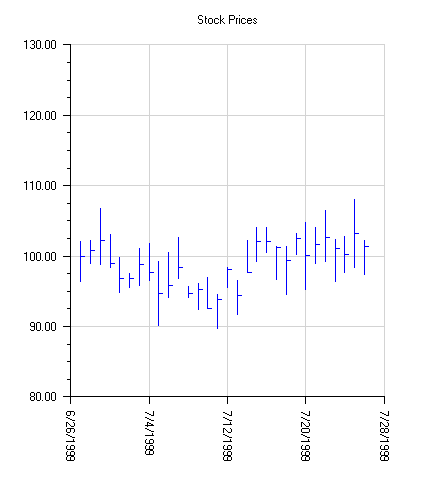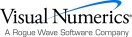## High-Low-Close Plot

High-Low-Close plots are used to show stock prices. They are created using the HighLowClose class.

The markers can be formatted using the attribute MarkerColor.

In a high-low-close plot the vertical line represent`s the high and low values. The close value is represented by a “tick” to the right. The open value, if present, is represented by a tick to the left.

The HighLowClose.SetDateAxis method will configure the x-axis for dates. This turns off autoscaling of the axis.

### Example

In this example, random security prices are computed in the CreateData method. The time axis is prepared by calling HighLowClose.SetDateAxis.using Imsl.Chart2D;

using System;

using System.Drawing;

public class SampleHighLowClose : FrameChart {

private double[] high, low, close;

public SampleHighLowClose() {

Chart chart = this.Chart;

AxisXY axis = new AxisXY(chart);

// Date is June 27, 1999

DateTime date = new DateTime(1999, 6, 27);

int n = 30;

CreateData(n);

//  Create an instance of a HighLowClose Chart

HighLowClose hilo = new HighLowClose(axis, date, high,

low, close);

hilo.MarkerColor = Color.Blue;

//  Set the HighLowClose Chart Title

chart.ChartTitle.SetTitle("Stock Prices");

// Setup the x axis

Axis1D axisX = axis.AxisX;

//  Set the text angle for the X axis labels

axisX.AxisLabel.TextAngle = 270;

// Setup the time axis

hilo.SetDateAxis("d");

// Turn on grid and make it light gray

axisX.Grid.IsVisible = true;

axisX.Grid.LineColor = Color.LightGray;

axis.AxisY.Grid.IsVisible = true;

axis.AxisY.Grid.LineColor = Color.LightGray;

}

private void CreateData(int n) {

high = new double[n];

low = new double[n];

close = new double[n];

Random r = new Random(123457);

for (int k = 0;  k < n;  k++) {

double f = r.NextDouble();

if (k == 0) {

close = 100;

} else {

close[k] = (0.95+0.10*f)*close[k-1];

}

high[k] = close[k]*(1+0.05*r.NextDouble());

low[k] = close[k]*(1-0.05*r.NextDouble());

}

}

public static void Main(string[] argv) {

System.Windows.Forms.Application.Run(

new SampleHighLowClose());

}

}Visual Numerics - Developers of IMSL and PV-WAVEhttp://www.vni.com/PHONE: 713.784.3131FAX:713.781.9260